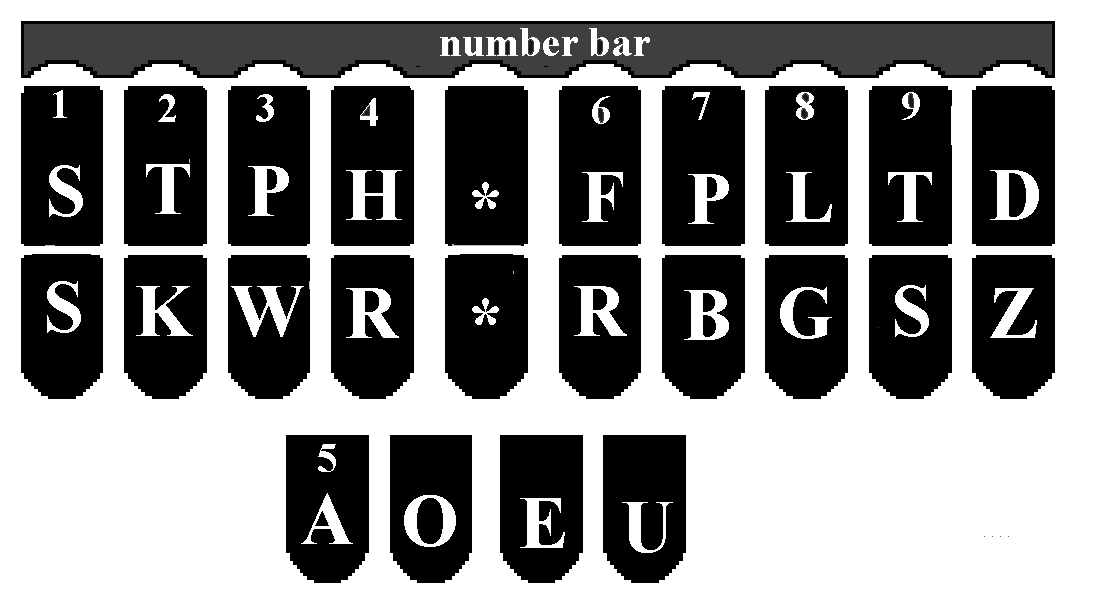# The Steno AlphabetI am about to release my first video teaching the steno alphabet. I’ve already posted videos on how to get set up to learn the alphabet before starting school, how to sit, how to type. But in just a week, I’ll be posting the first alphabet video. Search me on Youtube: Comrade Melody.

Remember to think of the steno alphabet in shapes, not letters. The words in parentheses are what they equal in Mark’s theory. The words outside of parentheses are what you would learn in any theory.

Initial side always has a hyphen AFTER the keys. Final side has the hyphen BEFORE the keys.

If you are learning the alphabet, copy and paste below this line into a notepad file and print, or you can just write everything below this line into your notebook:

————————————————————————————————————————–
INITIAL LEFT SIDE:
C = KR-
D = TK- (did)
F = TP- (if)
G = TKPW- (go)
J = SKWR- (judge)
K = K- (can)
L = L- (will)
M = PH- (many)
N = TPH- (in)
P = P- (.)
Q = KW-
R = R- (are)
S = S- (is)
T = T- (it)
V = SR- (have)
W = W- (with)
X = KP-
Y = KWR- (why)
Z = STKPWHR- (some theories do this S*)

FINAL RIGHT SIDE:
B = -B (be)
D = -D (-ed)
F = -F (of)
G = -G (-ing)
J = -PBLG (come)
K = -BG (being)
L = -L (-ly)
M = -PL (am)
N = -PB
P = -P (.)
R = -R (are)
S = -S (is)
T = -T (the)
V = *F (have)
X = -BGS (accident)
Z = -Z (-s)

VOWEL SOUNDS BOTTOM:
short A = A
short E = E (he)
short I = EU (I)
short O = O (on)
short U = U (you)

long A = AEU (a)
long E = AOE
long I = AOEU
long O = OE
long U = AOU

oy sound = OEU, as in the word “boy”
au sound = AU, as in the word “awe”
ou sound = OU, as in the word “out”
oo sound = AO, as in the word “too”
(AE = multisyllabic, as in “ready” RAED)

IMPORTANT:
SKP = and
P-P = .
W-B = ,
STPH = ?
-SD = said
-TZ = thoughts